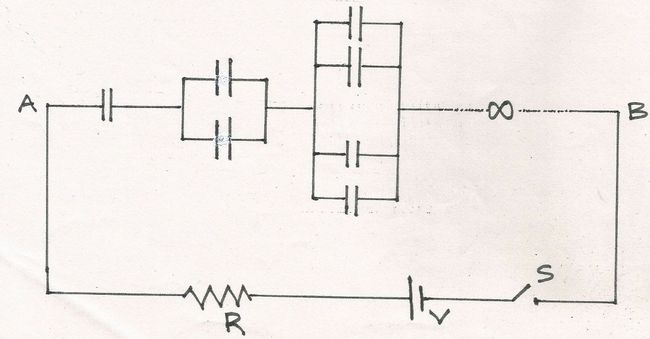# Infinite RC Circuit-2!In the given network shown, each capacitor is of capacitance $C$. The switch is closed at $t=0$.

Which of these statements is/are true?

1) Time Constant for the Circuit is $\frac{1}{2} RC$.
2) Left most capacitor will have highest charge.
3) Capacitors will have increasingly high potential differences across them as we move toward the right.
4) Total heat generated in the circuit is $\frac{1}{2} CV^2$

×

Problem Loading...

Note Loading...

Set Loading...